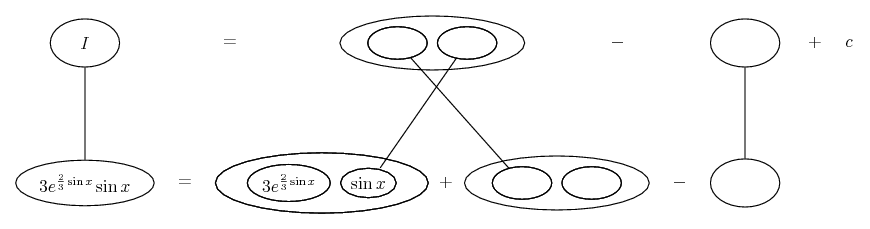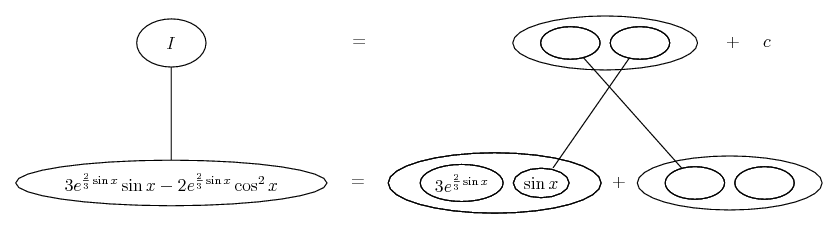# Thread: area of graph

1. ## area of graph

hi

need to find area of graph

$\displaystyle y=(2sinx-1)(sinx+2)exp(\frac{2}{3}sinx)$

i have limits from previous question $\displaystyle \frac{\pi}{6}$ and $\displaystyle \frac{5\pi}{6}$. i know i need to intergate this but i just can not do it. i have been trying to answer these question since 8am, could really do with some help.

many thanks

2. Multiply out the bracketted trig expressions and apply Pythag to convert the sin^2 to a cos^2. So you can split the integrand into two terms,

$\displaystyle 3 e^{\frac{2}{3}sin x}sin x - 2 e^{\frac{2}{3}sin x}cos^2 x$

Start on the second term by parts, and you should find a use for the first...

Edit:

(or even easier, the other way round, d'oh!)

Just in case a picture helps...... where... is the product rule, straight continuous lines differentiating downwards with respect to x. So integration by parts, if you want to do it with balloons (but of course!) is a matter of filling out the product-rule shape usefully, but starting in one of the bottom corners instead of (as happens in differentiation) the top one. So normally you have to subtract something (which becomes a new integration problem) to keep the lower equals sign valid. But in this case we strike lucky because the right fork of the product-rule shape turns out to be the term we put to the side earlier. So we can just bring it back in to play...__________________________________________

Don't integrate - balloontegrate!

http://www.ballooncalculus.org/forum/top.php

Draw balloons with LaTeX: http://www.ballooncalculus.org/asy/doc.html

#### Search Tags

area, graph